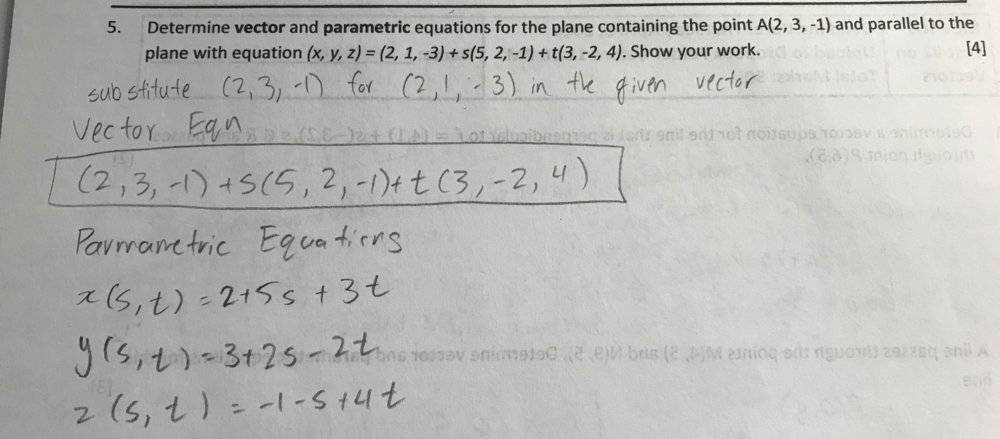# Calculus and Vectors - Determining Vector and Parametric Equations

• ttpp1124

#### ttpp1124

Homework Statement
Determine vector and parametric equations for the plane containing the point A(2, 3, -1) and parallel to the plane with equation (x, y, z) = (2, 1, -3) + s(5, 2, -1) + t(3, -2, 4).

Can someone confirm?
Relevant Equations
n/a#### Attachments

Homework Statement:: Determine vector and parametric equations for the plane containing the point A(2, 3, -1) and parallel to the plane with equation (x, y, z) = (2, 1, -3) + s(5, 2, -1) + t(3, -2, 4).

Can someone confirm?
Relevant Equations:: n/a

View attachment 260205
Comment: Your vector equation isn't an equation. An equation always has '=' in it.
Can you check these for yourself? Does your plane contain the point A(2, 3, -1)? Since your plane is parallel to the given plane, it should contain the same two vectors as the given plane.

Comment: Your vector equation isn't an equation. An equation always has '=' in it.
Can you check these for yourself? Does your plane contain the point A(2, 3, -1)? Since your plane is parallel to the given plane, it should contain the same two vectors as the given plane.

To obtain a plane parallel to P0 and passing through the point A(2,3,−1), all we need to do is add A as a vector (I dropped the coordinates for brevity) to all the points of P0. This gives us the plane

(𝑥,𝑦,𝑧)=𝐴+𝑠(5,2,−1)+𝑡(3,−2,4)

Last edited by a moderator:
To obtain a plane parallel to P0 and passing through the point A(2,3,−1), all we need to do is add A as a vector (I dropped the coordinates for brevity) to all the points of P0. This gives us the plane

(𝑥,𝑦,𝑧)=𝐴+𝑠(5,2,−1)+𝑡(3,−2,4)
Yes,, I understand how you got the equation, but I was responding to your request for someone to verify that your work was correct. Here's what I said before:
Can you check these for yourself? Does your plane contain the point A(2, 3, -1)? Since your plane is parallel to the given plane, it should contain the same two vectors as the given plane.
One of the things I've always liked about mathematics, especially at somewhat higher levels, is that it's not difficult to verify your work for yourself. What I said above was how you can do this.